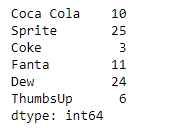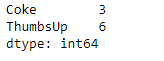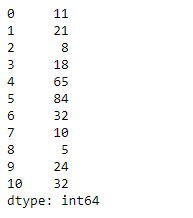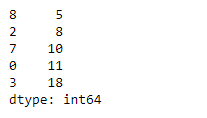# Python | Pandas Series.nsmallest()

• Last Updated : 11 Feb, 2019

Pandas series is a One-dimensional ndarray with axis labels. The labels need not be unique but must be a hashable type. The object supports both integer- and label-based indexing and provides a host of methods for performing operations involving the index.

Pandas` Series.nsmallest()` function return the n smallest element from the underlying data in the given series object.

Attention geek! Strengthen your foundations with the Python Programming Foundation Course and learn the basics.

To begin with, your interview preparations Enhance your Data Structures concepts with the Python DS Course. And to begin with your Machine Learning Journey, join the Machine Learning - Basic Level Course

Syntax: Series.nsmallest(n=5, keep=’first’)

Parameter :
n : Return this many ascending sorted values.
keep : {‘first’, ‘last’, ‘all’}, default ‘first’

Returns : Series

Example #1: Use `Series.nsmallest()` function to return the first n smallest element from the given series object.

 `# importing pandas as pd``import` `pandas as pd`` ` `# Creating the Series``sr ``=` `pd.Series([``10``, ``25``, ``3``, ``11``, ``24``, ``6``])`` ` `# Create the Index``index_ ``=` `[``'Coca Cola'``, ``'Sprite'``, ``'Coke'``, ``'Fanta'``, ``'Dew'``, ``'ThumbsUp'``]`` ` `# set the index``sr.index ``=` `index_`` ` `# Print the series``print``(sr)`

Output :Now we will use `Series.nsmallest()` function to find the first 2 smallest value in the given series object.

 `# return the first 2 of the smallest``# element``result ``=` `sr.nsmallest(n ``=` `2``)`` ` `# Print the result``print``(result)`

Output :As we can see in the output, the `Series.nsmallest()` function has successfully returned the first 2 smallest value in the given series object.

Example #2: Use `Series.nsmallest()` function to return the first n smallest element from the given series object.

 `# importing pandas as pd``import` `pandas as pd`` ` `# Creating the Series``sr ``=` `pd.Series([``11``, ``21``, ``8``, ``18``, ``65``, ``84``, ``32``, ``10``, ``5``, ``24``, ``32``])`` ` `# Print the series``print``(sr)`

Output :Now we will use `Series.nsmallest()` function to find the first 5 smallest value in the given series object.

 `# return the first 5 of the smallest``# element``result ``=` `sr.nsmallest(n ``=` `5``)`` ` `# Print the result``print``(result)`

Output :As we can see in the output, the `Series.nsmallest()` function has successfully returned the first 5 smallest value in the given series object.

My Personal Notes arrow_drop_up